### Chemical and Process Engineering Resources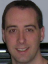## Pneumatic Conveying Design

Nov 08 2010 01:00 PM | Chris Haslego in Bulk SolidsNomenclature

 Vg Gas velocity [ft/s] rg Gas density [lbs/ft3] W Solids mass velocity [lbs/s ft2] Vp Particle velocity [ft/s] f Fanning friction factor L Equivalent length of pipeline [ft] D Pipe inside diameter [ft] g Acceleration due to gravity [32.2 ft/s2] gc Constant [32.174 ft-lb/lb s2] K Friction multiplier for the solids conveyed R Solids to gas mass flow ration [lb/lb] Z Elevation change in pipe line [ft]

Early is their work, Zenz and Othmer used the following equations to described the pressure losses in horizontal and vertical pipes: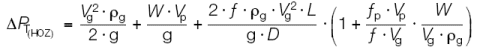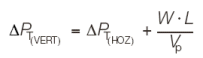It was later concluded that the term (fp Vp/f Vg) should be replaced by the constant K (friction multiplier for the solids conveyed) because the term was dependent upon the physical properties of the solids being conveyed. The value for K must be back-calculated from actual pressure drop data from an existing system or it must be determined experimentally in plant tests. This lead to the following equation for the pressure drop for the solids in the system.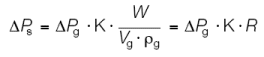Thus, the equation used for the total system pressure loss (Base Equation)Â is: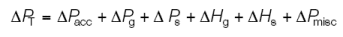where:

 DPT Total pressure loss in the system DPacc Pressure loss due to acceleration of the solids from their "at rest" condition at the pick up point. DPg Frictional pressure loss of the gas DPs Frictional pressure loss of the solids DHg Elevation pressure loss of the gas DHs Elevation pressure loss of the solids DPmisc Pressure losses from miscellaneous equipment

## 0 Comments

### Forum Quick Links

•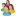Industrial Forum

•Student Forum

•Refining Forum

•Simulation Forum

•Relief Devices Forum

•Tank Venting Forum

• ### Article Navigation

•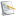Separation Technology

•Calculations and Tips

•Fluid Flow

•Heat Transfer

•Maintenance and Repair

•Utilities

•Safety and Pressure Relief

•Bulk Solids

•Process and Reactions

•Energy

•Other Topics

•For Students

•Physical Properties

•Archived Articles